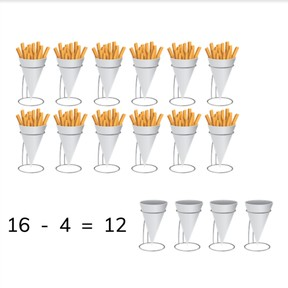Recognizing subtraction problems to 20

# Recognizing subtraction problems to 208,000 schools use Gynzy92,000 teachers use Gynzy1,600,000 students use Gynzy

## General

Students learn to match images to subtraction problems to 20.

1.OA.C.6
1.OA.C

## Relevance

Discuss with students that it is useful to use an image to determine what a subtraction problem is. You learn how subtraction works and are better able to calculate.

## Introduction

Show the white and red beads on the interactive whiteboard. Ask students to count how many white and red beads there are. Ask students if they know any clever ways to count. Say that one way to do so is to cross off the beads as you count them, so you don't miss any or count any twice. Next, show the image of the bears and count the bears together.

## Development

Show the image of the cans on the interactive whiteboard. Some of the cans are whole, and some are crushed. First count the total number of cans. Then count the total number of crushed cans. There are 20 cans in total, and 6 that are crushed. The 6 crushed cans are taken away. The subtraction problem that is represented is 20 - 6. Discuss all the variations of the subtraction problems shown by the cans and crushed cans. Next show the tree with apples. Count the apples- there are 19. Then count the apples that have fallen off the tree- there are 5. Emphasize to students that the - (minus sign) shows that something is being taken away and that the second number (subtrahend) is being taken away from the total, or first number (minuend). Repeat to students that the first number(minuend) in a subtraction problem is always the total, and the second number (subtrahend) is what is being taken away from the first number.
Next show a collection of paint tubes of which some have been emptied. Ask students if they can determine the subtraction problem shown by this image. Erase the grey boxes to show the answer. Students are given another image and asked to determine the subtraction problem of that image.
Next, using the image of the apples, discuss with students that when you have determined what the subtraction problem is, that you can also find the difference. You do so by taking the second number (subtrahend) away from the first number (minuend). The difference is what is left over. If you take away the apples that have fallen from the tree, you know how many apples you have left. Practice this with the example of the plates.

Check that students can recognize subtraction problems to 20 by asking the following questions:
- How do you know which numbers to use to form a subtraction problem?
- What must you do if you want to know how many you have left after taking one number away from another?
- What does the first number (minuend) of a subtraction problem mean?
- How do you know what the second number (subtrahend) of a subtraction problem is?

## Guided practice

Students first practice recognizing subtraction problems and must match a subtraction problem to a given image. They must then write out the subtraction problem shown by an image, and finally must give not only the subtraction problem, but also the difference of the given image.

## Closing

Discuss with students that it is important to be able to use an image to determine what a subtraction problem is, to help support your subtraction. Show a problem on the interactive whiteboard and have students drag the leaves so that they match the given subtraction problem. Ask students to draw a flower which has the correct number of petals on their own sheets of paper and then to hold them up in the air so they can be checked. Finally, drag the petals on the interactive whiteboard.

## Teaching tips

Students who have difficulty recognizing subtraction in an image can make use of MAB blocks or other manipulatives. Have them set out the total number of blocks and then practice taking away blocks (as represented by the second number- the subtrahend). Have them count how many are left.

## Instruction materials

Optional: MAB blocks or other manipulatives

### The online teaching platform for interactive whiteboards and displays in schools

• Save time building lessons

• Manage the classroom more efficiently

• Increase student engagement